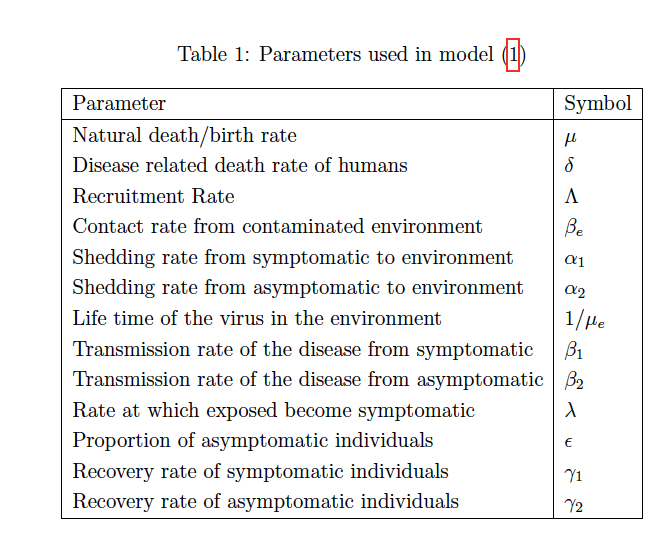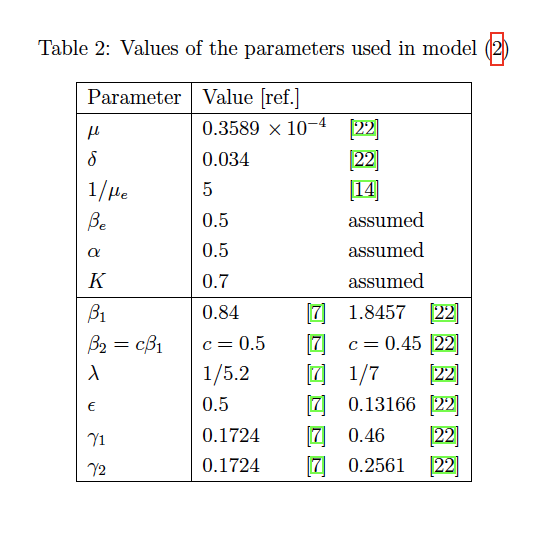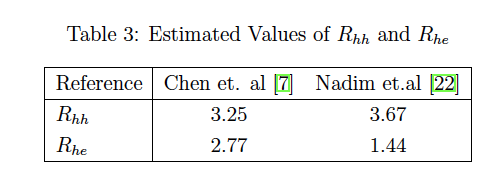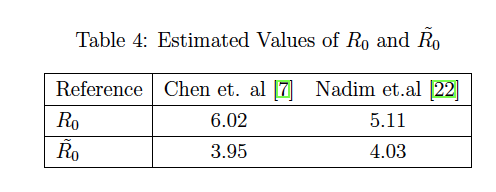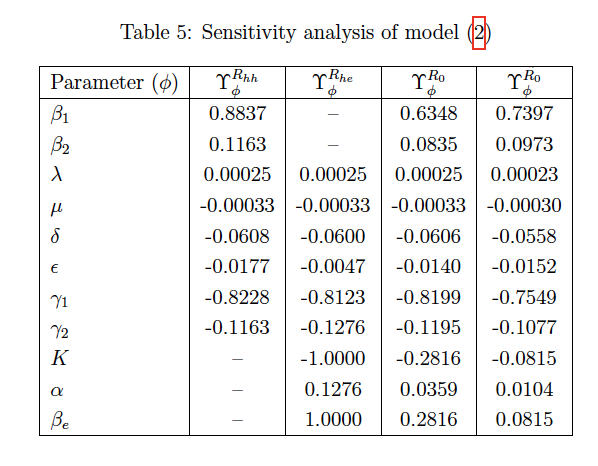# Investigating the Role of Environmental Transmission in COVID-19 Dynamics: A Mathematical Model Based Study

## Abstract

A mathematical model with environmental transmission has been proposed and analyzed to investigate its role in the transmission dynamics of the ongoing COVID-19 outbreak. Two expressions for the basic reproduction number R0 have been analytically derived using the next generation matrix method. The two expressions composed of a combination of two terms related to human to human and environment to human transmissions. The value of R0 has been calculated using estimated parameters corresponding to two datasets. Sensitivity analysis of the reproduction number to the corresponding model parameters has been carried out. Existence and stability analysis of disease free and endemic equilibrium points have been presented in relation with the obtained expressions of R0. Numerical simulations to demonstrate the effect of some model parameters related to environmental transmission on the disease transmission dynamics have been carried out and the results have been demonstrated graphically.

## Full Text

This preprint is available for download as a PDF.

## Tables